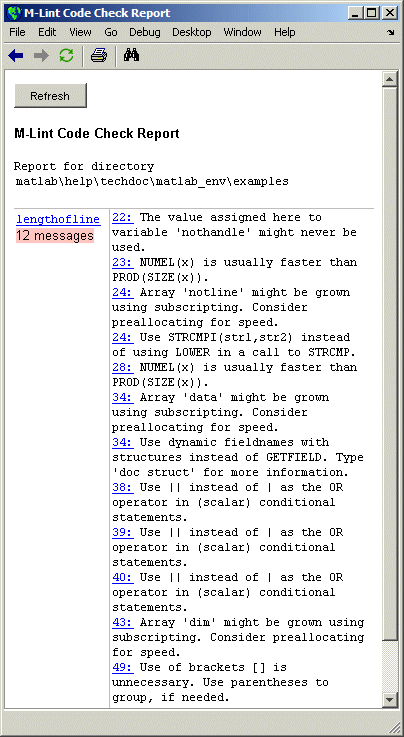Desktop Tools and Development EnvironmentMaking Changes Based on M-Lint Messages

For information on how to correct the potential problems presented by M-Lint, use the following resources:

Example Using M-Lint Messages to Improve Code

An example file, `lengthofline.m`, is included with MATLAB in `\$matlabroot/matlab/help/techdoc/matlab_env/examples`.

To run the M-Lint Code Check Report for `lengthofline.m`, use the Current Directory browser to navigate to the `\$matlabroot/matlab/help/techdoc/matlab_env/examples` directory. Select the M-Lint Code Check Report from the list of reports on the toolbar. Note that `lengthofline.m` is not on the MATLAB path by default. You can run the file or open it in the Editor/Debugger when `\$matlabroot/matlab/help/techdoc/matlab_env/examples` is the current directory.

The M-Lint Code Check Report appears, with its list of messages suggesting improvements you can make to `lengthofline.m`.The following table describes each message and demonstrates a way to change the file, based on the message.

Message -- -- Code
Explanation and Updated Code
`22: The value assigned here to variable 'nothandle' might never be used.`
-- -- -- -- -- -- -- -- -- -- -- -- -- -- -- -- --
`22 nothandle = ~ishandle(hline);`
`23 for nh = 1:prod(size(hline))`
`24 notline(nh) = ~ishandle(hline(nh)) ...`
In line 22, `nothandle` is assigned a value, but `nothandle` is probably not used anywhere after that in the file. The line might be extraneous and you could delete it. But it might be that you actually intended to use the variable, which is the case for the `lengthofline` example. Update line 24 to use `nothandle`, which is faster than computing `~ishandle` for each iteration of the loop, as shown here.
`nothandle = ~ishandle(hline);`
`for nh = 1:numel(hline)`
`notline(nh) = nothandle(nh) ...`
`23: NUMEL(x) is usually faster than PROD(SIZE(x)).`
-- -- -- -- -- -- -- -- -- -- -- -- -- -- -- -- --
`23 for nh = 1:prod(size(hline))`
While `prod(size(x))` returns the number of elements in a matrix, the `numel` function was designed to do just that, and therefore is usually more efficient. Type `doc numel` to see the `numel` reference page. Change the line to
for nh = 1:numel(hline)
`24: Array 'notline' might be grown using subscripting. Consider preallocating for speed.`
-- -- -- -- -- -- -- -- -- -- -- -- -- -- -- -- --
`22 nothandle = ~ishandle(hline);`
`23 for nh = 1:numel(hline)`
`24 notline(nh) = ~ishandle(hline(nh)) ...`
When you increase the size of an array within a loop, it is inefficient. Before the loop, preallocate the array to its maximum size to improve performance. For more information, see Preallocating Arrays in the MATLAB Programming documentation. In the example, add a new line to preallocate `notline` before the loop.
`notline = false(size(hline));`
`for nh = 1:numel(hline)`
`notline(nh) = nothandle(nh) ...`
`24: Use STRCMPI(str1,str2) instead of using LOWER in a call to STRCMP.`
-- -- -- -- -- -- -- -- -- -- -- -- -- -- -- -- --
`24 notline(nh)=~ishandle(hline(nh)) || ~strcmp('line',lower(get(hline(nh),'type')));`
While
strcmp
('line',lower(get(hline(nh)'type'))
converts the result of the `get` function to a lowercase string before doing the comparison, the `strcmpi` function ignores the case while performing the comparison, with advantages that include more efficiency. Change the line to
`notline(nh) = nothandle(nh) || ~strcmpi('line',get(hline(nh),'type'));`
`28: NUMEL(x) is usually faster than PROD(SIZE(x)).`
-- -- -- -- -- -- -- -- -- -- -- -- -- -- -- -- --
`28 for nl = 1:prod(size(hline))`
See the same message and explanation reported for line 23. Change the line to
`for nl = 1:numel(hline)`
`34: Array 'data' might be grown using subscripting. Consider preallocating for speed.`
-- -- -- -- -- -- -- -- -- -- -- -- -- -- -- -- --
`33 for nd = 1:length(fdata)`
`34 data{nd} =getfield(flds,fdata{nd});`
See the same message and explanation reported for line 24. Add this line before the loop
`data = cell(size(fdata)); `
`34: Use dynamic fieldnames with structures instead of GETFIELD. Type 'doc struct' for more information.`
-- -- -- -- -- -- -- -- -- -- -- -- -- -- -- -- --
`34 data{nd} =getfield(flds,fdata{nd});`
You can access a field in a structure as a variable expression that MATLAB evaluates at run-time. This is more efficient than using `getfield`. For more information, type `doc struct` to see the reference page for structures, or see Using Dynamic Field Names in the MATLAB Programming documentation. Change the line to
`data{nd} = flds.(fdata{nd});`
`38: Use || instead of | as the OR operator in (scalar) conditional statements.`
`39: Use || instead of | as the OR operator in (scalar) conditional statements.`
`40: Use || instead of | as the OR operator in (scalar) conditional statements.`
-- -- -- -- -- -- -- -- -- -- -- -- -- -- -- -- --
`38 if isempty(data{3}) | ...`
`39 (length(unique(data{1}(:)))==1 | ...`
`40 length(unique(data{2}(:)))==1 | ...`
`41 length(unique(data{3}(:)))==1)`
While | (the elementwise logical `OR` operator) performs the comparison correctly, use the || (short circuit `OR` operator) for efficiency. For details, see Logical Operators in the MATLAB Programming documentation. Change the lines to
`if isempty(data{3}) || ...`
`(length(unique(data{1}(:)))==1 || ...`
`length(unique(data{2}(:)))==1 || ...`
`length(unique(data{3}(:)))==1)`
`43: Array 'dim' might be grown using subscripting. Consider preallocating for speed.`
`43 dim(nl) = 2;`
See the same message and explanation reported for line 24. Before the first line of the loop
`29 for nl = 1:numel(hline)`
`dim = len;`
`49: Use of brackets [] is unnecessary. Use parentheses to group, if needed.`
-- -- -- -- -- -- -- -- -- -- -- -- -- -- -- -- --
`49 len(nl) =sum([sqrt(dot(temp',temp'))]);`
For more information about the use of brackets and parentheses, see the Special Characters reference page. In this example, remove the brackets because they are not needed. They add processing time because MATLAB concatenates unnecessarily. Change the line to
`len(nl) = sum(sqrt(dot(temp',temp')));`You can view the M-file with all of these changes. Navigate to the `\$matlabroot/matlab/help/techdoc/matlab_env/examples` directory and open `lengthofline2.m`.M-Lint Graphical User Interface (GUI) Profiling for Improving Performance